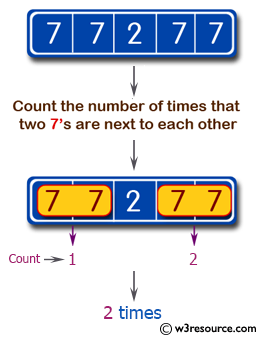﻿ Swift Basic Programming Exercise: Count the number of times that two 7's are next to each other in a given array of integers - w3resource# Swift Basic Programming Exercise: Count the number of times that two 7's are next to each other in a given array of integers

## Swift Basic Programming: Exercise-27 with Solution

Write a Swift program to count the number of times that two 7's are next to each other in a given array of integers.

Pictorial Presentation:Sample Solution:

Swift Code:

``````func array77(_ input: [Int]) -> Int {
var ctr = 0
for (index, number) in input.enumerated() {
let nextIndex = index + 1

if nextIndex < input.endIndex && number == 7 && (input[nextIndex] == 7 )
{
ctr += 1
}
}
return ctr
}

print(array77([7, 7, 3]))
print(array77([7, 7, 2, 7, 7]))
print(array77([7, 5, 2, 7]))
```
```

Sample Output:

```1
2
0
```

Swift Programming Code Editor:

Improve this sample solution and post your code through Disqus

What is the difficulty level of this exercise?

﻿